# Introduction

This document demonstrates how to use the systematic variation estimation methods of the hettx package. First load the package:

library( hettx )

This package includes code to make synthetic data, which can be useful for simulation studies and illustrations. Here we make a dataset with 3 covariates that we will use to illustrate the function calls. For this dataset, the first two variables have a systematic relationship with treatment impact, and the third is good for adjustment for increasing power:

df = make.randomized.dat( 10000, gamma.vec=c(1,1,1,2), beta.vec=c(-1,-1,1,0) )
str( df )
## 'data.frame':    10000 obs. of  8 variables:
##  $A : num 0.7188 0.0832 0.4373 0.8153 0.1497 ... ##$ B   : num  1.142 0.478 -2.093 -0.715 0.792 ...
##  $C : num -0.317 -0.439 -2.023 -0.887 0.955 ... ##$ Y.0 : num  1.467 -0.204 -3.96 -1.868 4.44 ...
##  $Y.1 : num 0.89 -0.81 -7.49 -4.4 4.08 ... ##$ tau : num  -0.577 -0.605 -3.531 -2.531 -0.357 ...
##  $Z : num 1 0 1 0 1 1 1 0 1 0 ... ##$ Yobs: num  0.89 -0.204 -7.491 -1.868 4.083 ...

## Basic estimation

The function estimate_systematic is our core estimator that implements our various methods:

rs = estimate_systematic( Yobs ~ Z,  interaction.formula = ~ A + B, data=df )
summary(rs)
##
## Systematic Estimation Regression of Heterogeneous Treatment Effects: RI-Unadjusted
##
## Call:
## estimate_systematic(formula = Yobs ~ Z, data = df, interaction.formula = ~A +
##     B)
##
## Coefficients:
## (Intercept)            A            B
##     -0.9165      -1.1582       0.9924
##
## Variance-Covariance Matrix:
##              (Intercept)             A             B
## (Intercept) 0.0052191838  0.0006408565  0.0002679515
## A           0.0006408565  0.0082436289 -0.0027826841
## B           0.0002679515 -0.0027826841  0.0091878902
##
## Chi-squared test for systematic variation: X^2=206.57; p=0.000
##
## Details: ATE = -0.920 +/- 0.142     SD(Y(0)) = 3.520   SD(Y(1)) = 3.565

The arguments are observed outcome, observed treatment assignment, and what variables to find a systematic relationship to, expressed as a formula using the tilde notation (categorical covariates will be automatically converted). The output give coefficients for the model for individual systematic treatment effects. In the above, our model of effects is $$\tau_i = \beta_0 + \beta_1 A_i + \beta_2 B_i$$.

We can obtain our standard errors and variance-covariance matrix for our estimators that comes from the design-based theory:

vcov( rs )
##              (Intercept)             A             B
## (Intercept) 0.0052191838  0.0006408565  0.0002679515
## A           0.0006408565  0.0082436289 -0.0027826841
## B           0.0002679515 -0.0027826841  0.0091878902
SE( rs )
## (Intercept)           A           B
##  0.07224392  0.09079443  0.09585348

and confidence intervals (using the normal approximation)

confint( rs )
##                  2.5 %     97.5 %
## (Intercept) -1.0580920 -0.7749010
## A           -1.3361352 -0.9802276
## B            0.8044838  1.1802226

OLS uses the empirical covariance matrix $$\widehat{S}_{xx}$$ (Sxx.hat) for each treatment arm rather than the known Sxx:

M.ols.ours = estimate_systematic( Yobs ~ Z, ~ A + B, data=df, method="OLS" )
summary(M.ols.ours)
##
## Systematic Estimation Regression of Heterogeneous Treatment Effects: OLS-Unadjusted
##
## Call:
## estimate_systematic(formula = Yobs ~ Z, data = df, interaction.formula = ~A +
##     B, method = "OLS")
##
## Coefficients:
## (Intercept)            A            B
##     -0.9395      -1.0868       1.0503
##
## Variance-Covariance Matrix:
##               (Intercept)             A             B
## (Intercept)  1.557823e-03 -8.856266e-07  2.126964e-06
## A           -8.856266e-07  2.133263e-03 -1.135415e-03
## B            2.126964e-06 -1.135415e-03  2.165900e-03
##
## Chi-squared test for systematic variation: X^2=696.30; p=0.000
##
## Details: ATE = -0.920 +/- 0.142     SD(Y(0)) = 3.520   SD(Y(1)) = 3.565
M.ols.ours$beta.hat ## (Intercept) A B ## -0.9394513 -1.0868438 1.0503174 Simple interaction-based OLS approach, as a comparison: M0 = lm( Yobs ~ (A+B) * Z, data=df ) M0 ## ## Call: ## lm(formula = Yobs ~ (A + B) * Z, data = df) ## ## Coefficients: ## (Intercept) A B Z A:Z ## 0.9436 1.7303 1.6019 -0.9395 -1.0868 ## B:Z ## 1.0503 There are no differences up to machine precision: M.ols.ours$beta - coef(M0)[4:6]
##   (Intercept)             A             B
## -5.107026e-15 -4.218847e-15  1.065814e-14

The model-adjusted estimator is used automatically if you give two formula, one for the treatment model and one for the control adjustment model.

estimate_systematic( Yobs ~ Z, interaction.formula = ~ A + B,
control.formula = ~ C, data=df )
##
## Coefficients:
## (Intercept)            A            B
##     -1.0065      -1.1689       0.9894
##
## Variance-Covariance Matrix:
##              (Intercept)             A             B
## (Intercept) 0.0014574829  0.0001869543  0.0001395329
## A           0.0001869543  0.0081761768 -0.0028067889
## B           0.0001395329 -0.0028067889  0.0091776619
##
## Chi-squared test for systematic variation: X^2=209.22; p=0.000

These formula can use the same covariates. Here we also adjust for the covariates used in our treatment model:

rsA2 = estimate_systematic( Yobs ~ Z,  ~ A + B, ~ A + B + C, data=df )
coef( rsA2 )
## (Intercept)           A           B
##  -0.9912985  -1.1627770   0.9900589

We can also adjust for additional covariates using the OLS implementation:

rsB = estimate_systematic( Yobs ~ Z,  ~ A + B, ~ C, data=df, method = "OLS" )
coef( rsB )
## (Intercept)           A           B
##   -1.029519   -1.097512    1.047393
rsB2 = estimate_systematic( Yobs ~ Z,  ~ A + B, ~ A + B + C, data=df, method = "OLS" )
coef( rsB2 )
## (Intercept)           A           B
##   -1.014279   -1.091394    1.047997

As a comparison, using lm() we have

rsB.lm = lm( Yobs ~ Z * (A+B) + C, data=df )
coef( rsB.lm )
## (Intercept)           Z           A           B           C         Z:A
##   0.9937309  -0.9909066   1.0090723   1.0126931   2.0062109  -1.0344741
##         Z:B
##   1.0029401
cbind( C.only=coef( rsB ), ABC=coef( rsB2 ), lmC=coef( rsB.lm )[c(2,6,7)])
##                C.only       ABC        lmC
## (Intercept) -1.029519 -1.014279 -0.9909066
## A           -1.097512 -1.091394 -1.0344741
## B            1.047393  1.047997  1.0029401

Note that the model adjustment approach is not the same as including a term as a control variable in a linear regression (and you can do both).

# Oracle estimator (for simulations and verification of formulae)

If we know all potential outcomes, we can calculate the exact beta for the sample. (This is useful for simulation studies.) We can also get the true SE, which is why we pass a sample treatment vector (so it can calculate proportion treated, under the assumption of simple random assignment):

Moracle = estimate_systematic( Y.1 + Y.0 ~ Z, ~ A + B, data=df )
summary(Moracle)
##
## Systematic Estimation Regression of Heterogeneous Treatment Effects: Oracle RI
##
## Call:
## estimate_systematic(formula = Y.1 + Y.0 ~ Z, data = df, interaction.formula = ~A +
##     B)
##
## Coefficients:
## (Intercept)            A            B
##          -1           -1            1
##
## Variance-Covariance Matrix:
##              (Intercept)            A             B
## (Intercept) 0.0050504890  0.000531448  0.0004005199
## A           0.0005314480  0.007735527 -0.0024893923
## B           0.0004005199 -0.002489392  0.0088302172
##
## Chi-squared test for systematic variation: X^2=NA; p=NA
SE( Moracle )
## (Intercept)           A           B
##  0.07106679  0.08795184  0.09396924

It will give the same results regardless of $$Z$$ assuming the total number of units remains the same.

# Looking at $$R^2$$

We can look at treatment effect explained. We will look at two scenarios, one with no ideosyncratic variation on top of the systematic variation, and one with a substantial amount. We will plot the R2 sensitivity curves for each on top of each other.

df = make.randomized.dat( 1000, beta.vec=c(-1,1,1) )
rs = estimate_systematic( Yobs ~ Z, ~ A + B, data=df, method="OLS" )
r2 = R2( rs )
r2
##
##  R2 for Systematic Estimation Regression of Heterogeneous Treatment Effects (ITT)
##
## R2 Estimates:
##   R2 Lower Bound R2 Lower Bound (Sharp) R2 Upper Bound
## 1         0.3195                 0.4811         0.9956
##
## Variance Estimates:
##  Systematic Treatment Effect Variation: 2.994
##  Idiosyncratic Treatment Effect Variation: 0.0131 -- 6.378 (3.2293 Sharp)
##  Total Treatment Effect Variation: 3.0071 -- 9.372 (6.2232 Sharp)

And now our DGP with lots of idiosyncratic variation:

df = make.randomized.dat( 1000, beta.vec=c(-1,1,1), ideo.sd=3 )
rs = estimate_systematic( Yobs ~ Z, ~ A + B, data=df, method="OLS" )
r2b = R2( rs )
r2b    
##
##  R2 for Systematic Estimation Regression of Heterogeneous Treatment Effects (ITT)
##
## R2 Estimates:
##   R2 Lower Bound R2 Lower Bound (Sharp) R2 Upper Bound
## 1         0.1598                 0.2444         0.5321
##
## Variance Estimates:
##  Systematic Treatment Effect Variation: 3.7259
##  Idiosyncratic Treatment Effect Variation: 3.2769 -- 19.5971 (11.5197 Sharp)
##  Total Treatment Effect Variation: 7.0028 -- 23.323 (15.2457 Sharp)

Plot our results:

plot( r2 )
plot( r2b, ADD=TRUE, col="green" )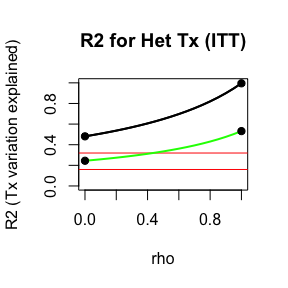And here is a case where we have 100% systematic variation along a single variable.

df = make.randomized.dat( 1000, beta.vec=c(-1,1,0) )
rs = estimate_systematic( Yobs ~ Z, ~ A + B, data=df, method="OLS" )
r2 = R2( rs )
r2    
##
##  R2 for Systematic Estimation Regression of Heterogeneous Treatment Effects (ITT)
##
## R2 Estimates:
##   R2 Lower Bound R2 Lower Bound (Sharp) R2 Upper Bound
## 1         0.1839                 0.3072          0.993
##
## Variance Estimates:
##  Systematic Treatment Effect Variation: 1.4597
##  Idiosyncratic Treatment Effect Variation: 0.0103 -- 6.4775 (3.2915 Sharp)
##  Total Treatment Effect Variation: 1.47 -- 7.9372 (4.7512 Sharp)
plot( r2 )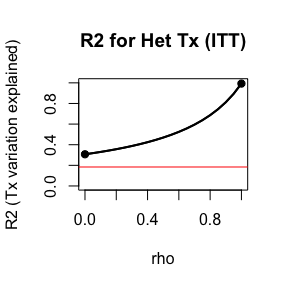See, we have 100% $$R^2_\tau$$, if we knew the true individual treatment effects:

plot( df$tau ~ df$A )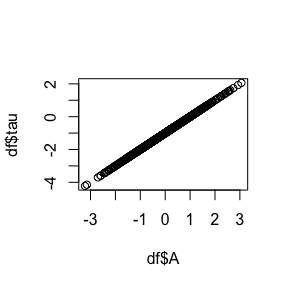## Comparing estimators

Here we look at how our ability to capture $$R^2_\tau$$ differs across different estimators. We have systematic effects for both $$A$$ and $$B$$, and $$C$$ is related to baseline outcomes but not impacts.

set.seed( 1020 )
df = make.randomized.dat( 1000, beta.vec=c(-1,1,1),
gamma.vec = c( 1, 2, 2, 1 ),
ideo.sd=1 )

rs = estimate_systematic( Yobs ~ Z, ~ A + B, data=df )
r2 = R2( rs )
plot( r2, col="green" )

rs = estimate_systematic( Yobs ~ Z, ~ A + B, ~ C, data=df )
r2 = R2( rs )
plot( r2, ADD=TRUE )

# adjusted + OLS
rs = estimate_systematic( Yobs ~ Z, ~ A + B, ~ C, data=df, method = "OLS" )
r2 = R2( rs )
plot( r2, ADD=TRUE, col="blue" )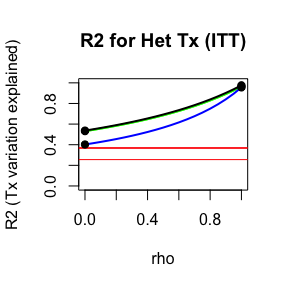# Treatment variation and non-compliance

Our estimators also work in non-compliance contexts (see paper for details). The story is analogous to the code above.

For this illustration we again generate some fake data using a provided function included with the package. This method takes a complier treatment heterogeniety model defined by beta:

beta = c(-1,6,0)
n = 10000

data = make.randomized.compliance.dat( n, beta.vec=beta )
names(data)
##   "A"    "B"    "C"    "Y.0"  "Y.1"  "tau"  "Z"    "Yobs" "S"    "D"

Our four observable groups defined by treatment assignment and take-up are as follows:

zd = with( data, interaction( Z, D, sep="-" ) )
boxplot( Yobs ~ zd, data=data, ylab="Yobs")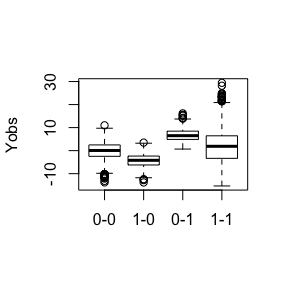The true relationships for the three latent groups are as follows:

par( mfrow=c(1,2), mgp=c(1.8,0.8,0), mar=c(3,3,0.5,0.5) )
plot( Y.1 - Y.0 ~ A, data=data, col=as.factor(data$S), pch=19, cex=0.5 ) plot( Y.1 - Y.0 ~ B, data=data, col=as.factor(data$S), pch=19, cex=0.5 )
legend( "topleft", legend=levels( as.factor( data$S ) ), pch=19, col=1:3 )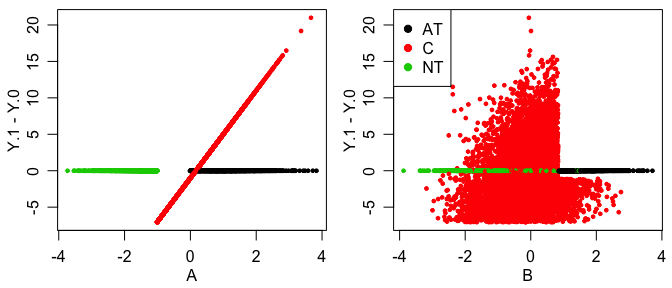(We see no impacts for the AT and the NT as required under the assumptions of noncompliance here.) In this scenario we have a moderate compiance rate, meaning not a particulary weak instrument: prop.table( table( data$S ) )
##
##     AT      C     NT
## 0.1571 0.6929 0.1500

## Estimating the effects

We use our same method, but by using the bar’’ notation, we can specify our treatment assigment $$Z$$ and our compliance status $$D$$ in our primary formula. Now our treatment variation formula is for the compliers (the always- and never-takers have no impact or variation).

rs = estimate_systematic( Yobs ~ D | Z, ~ A + B, data=data )
summary(rs)
##
## Systematic Estimation Regression of Heterogeneous Treatment Effects: LATE RI-RI
##
## Call:
## estimate_systematic(formula = Yobs ~ D | Z, data = data, interaction.formula = ~A +
##     B)
##
## Coefficients:
## (Intercept)            A            B
##     -1.0478       5.8345      -0.1873
##
## Variance-Covariance Matrix:
##             (Intercept)          A          B
## (Intercept) 0.008096388 0.01352146 0.01422525
## A           0.013521456 0.05316269 0.03072723
## B           0.014225251 0.03072723 0.03376611
##
## Chi-squared test for systematic variation: X^2=1431.94; p=0.000
rs$beta.hat ## (Intercept) A B ## -1.0477506 5.8345331 -0.1873088 SE( rs ) ## (Intercept) A B ## 0.08997993 0.23057036 0.18375556 We can get our R2 measure r2 = R2( rs ) r2 ## ## R2 for Systematic Estimation Regression of Heterogeneous Treatment Effects (LATE) ## ## R2 Estimates: ## R2 Lower Bound R2 Lower Bound (Sharp) ## Compliers 0.6880 0.8023 ## Noncompliers 0.0003 0.0004 ## Covariates and compliers 0.6881 0.8024 ## R2 Upper Bound ## Compliers 0.9770 ## Noncompliers 0.0005 ## Covariates and compliers 0.9770 ## ## Variance Estimates: ## Systematic Treatment Effect Variation for Compliers: 17.6422 ## Systematic Treatment Effect Variation for Strata: 0.0062 ## Total Systematic Treatment Effect Variation: 12.3131 ## Idiosyncratic Treatment Effect Variation for Compliers: 0.4153 -- 8.002 (4.3472 Sharp) ## Total Treatment Effect Variation: 12.6028 -- 17.8951 (15.3456 Sharp) ## ## Details: LATE = -0.172; ITT = -0.120; Proportion compliers = 0.698 plot( r2 )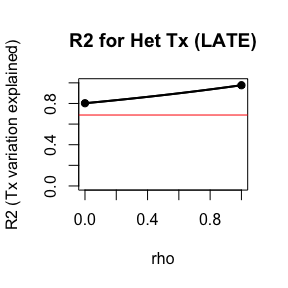## The 2SLS Approach Analogous to the OLS approach, above, we can use a 2SLS approach here. rs2SLS = estimate_systematic( Yobs ~ Z | D, ~ A + B, data=data, method="2SLS" ) summary(rs2SLS) ## ## Systematic Estimation Regression of Heterogeneous Treatment Effects: LATE RI-2SLS ## ## Call: ## estimate_systematic(formula = Yobs ~ Z | D, data = data, interaction.formula = ~A + ## B, method = "2SLS") ## ## Coefficients: ## (Intercept) A B ## -2.8306 9.0468 -0.6891 ## ## Variance-Covariance Matrix: ## (Intercept) A B ## (Intercept) 0.2204535 0.1049694 0.2992706 ## A 0.1049694 0.1454647 0.1740585 ## B 0.2992706 0.1740585 0.4491327 ## ## Chi-squared test for systematic variation: X^2=1113.08; p=0.000 SE( rs2SLS ) ## (Intercept) A B ## 0.4695247 0.3813984 0.6701736 Comparing our errors in estimation from the two approaches we have: err = rs$beta.hat - beta
err2SLS = rs2SLS\$beta.hat - beta
data.frame( SE.RI = SE( rs ), err.RI=err, SE.2SLS = SE( rs2SLS ), err.2SLS = err2SLS )
##                  SE.RI      err.RI   SE.2SLS   err.2SLS
## (Intercept) 0.08997993 -0.04775056 0.4695247 -1.8305835
## A           0.23057036 -0.16546692 0.3813984  3.0468213
## B           0.18375556 -0.18730879 0.6701736 -0.6891083